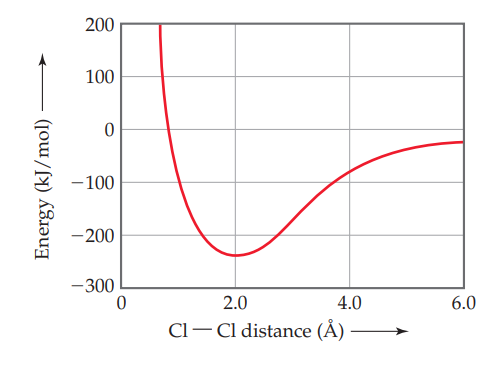×
Get Full Access to Chemistry: The Central Science - 14 Edition - Chapter 9 - Problem 9.5
Get Full Access to Chemistry: The Central Science - 14 Edition - Chapter 9 - Problem 9.5

×

# ?The following plot shows the potential energy of two Cl atoms as a function of the distance between them. (a) If the two atoms are very farISBN: 9780134414232 1274

## Solution for problem 9.5 Chapter 9

Chemistry: The Central Science | 14th Edition

• Textbook Solutions
• 2901 Step-by-step solutions solved by professors and subject experts
• Get 24/7 help from StudySoup virtual teaching assistantsChemistry: The Central Science | 14th Edition

4 5 1 391 Reviews
27
2
Problem 9.5

The following plot shows the potential energy of two Cl atoms as a function of the distance between them.

(a) If the two atoms are very far away from each other, what is their potential energy of interaction?

(b) We know that the $$\mathrm{Cl}_{2}$$ molecule exists. What is the approximate bond length and bond strength for the Cl– Cl bond in $$\mathrm{Cl}_{2}$$ from this graph?

(c) If the $$\mathrm{Cl}_{2}$$ molecule is compressed under higher and higher pressure, does the Cl–Cl bond become stronger or weaker?Text Transcription:

Cl_2

Step-by-Step Solution:

Step 1 of 5) Sodium fluoride has the sodium chloride structure with a coordination number of 6 for both cation and anion, as you might expect since NaF and NaCl are quite similar. Magnesium fluoride, however, has two anions for every cation, resulting in a tetragonal crystal structure called the rutile structure. The cation coordination number is still 6, but the fluoride coordination number is now only 3. In the scandium fluoride structure, there are three anions for every cation; the cation coordination number is still 6, but the fluoride coordination number has dropped to 2. As the cation/anion ratio goes down, there are fewer cations to surround each anion, and so the anion coordination number must decrease. The empirical formula of an ionic compound can be described quantitatively by the relationship. We need to count the number of anions in the unit cell of the sodium chloride structure, remembering that ions on the corners, edges, and faces of the unit cell are only partially inside the unit cell. We can apply the same approach to determine the number of cations in the unit cell. We can double-check our answer by writing the empirical formula to make sure the charges of the cations and anions are balanced. Because density is an intensive property, the density of the unit cell is the same as the density of a bulk crystal. To calculate the density we must divide the mass of the atoms per unit cell by the volume of the unit cell. To determine the volume of the unit cell, we need to estimate the length of the unit cell edge by first identifying the direction along which

Step 2 of 1

## Discover and learn what students are asking

Chemistry: The Central Science : Introduction:Matter, Energy, and Measurement
?Which of the following diagrams represents a chemical change? [Section 1.3]

Statistics: Informed Decisions Using Data : An Overview of Nonparametric Statistics
?Why is it appropriate to call nonparametric statistical procedures distribution-free procedures?

Unlock Textbook Solution